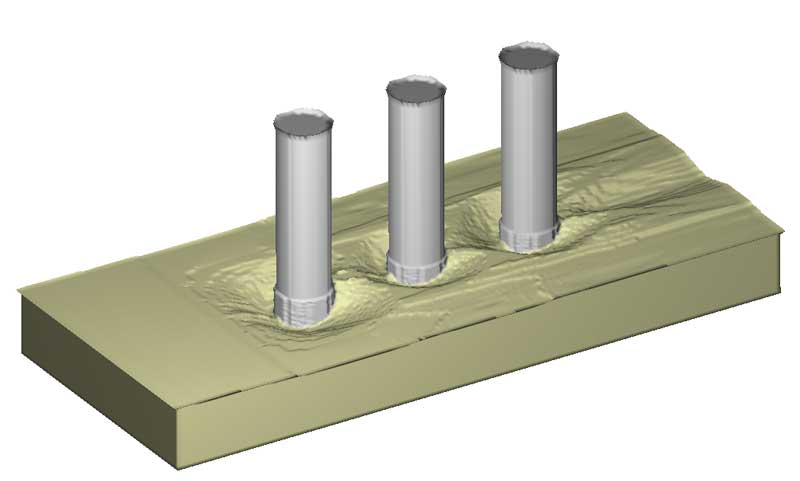# Sediment Scour

Fully-coupled with fluid dynamics, the sediment scour model in FLOW-3D simulates all the sediment transport processes of non-cohesive soil including bedload transport, suspended load transport, entrainment and deposition (Wei et al. 2014). It allows multiple sediment species with different properties such as grain size, mass density and critical shear stress. For example, medium sand, coarse sand and fine gravel can be categorize into three different species in a simulation. The model works for both 3D flows and 2D shallow water flows.

In the model, the packed bed of sediments is defined by one geometry component that can be composed of multiple subcomponents with different combination of sediment species. The packed bed is described by the FAVORTM technique using the area and volume fractions. In the mesh cells containing the bed interface, location, orientation and area of the interface are calculated and utilized to determine the bed shear stress, the critical Shields parameter, the erosion rate and the bedload transport rate. Bed shear stress in 3D turbulent flows is evaluated using the standard wall function with consideration of bed surface roughness that is proportional to the media grain sized50. For 2D shallow water flows, bed shear stress calculation follows the quadratic law where the drag coefficient is either user-defined or locally calculated using the water depth and the bed surface roughness.Figure 1. Flow at t=8 min

The model calculates bedload transport in each mesh cell containing the bed interface using the equation of Meyer-Peter and Muller (1948). A submesh method is employed to determine the amount of grains moving from the mesh cell into each mesh cell in its neighbor. Suspended sediment concentrations are obtained by solving the sediment transport equations. Computation of erosion considers sediment entrainment and deposition simultaneously. The lifting velocity of grains in entrainment is calculated using the equation of Winterwerp et al. (1992). The settling velocity in deposition is equal to the drift velocity of sediments for 3D flows but is calculated using an existing equation (Soulsby, 1997) for shallow water flows. A drift flux theory (Brethour and Hirt, 2009) is used to compute the drift velocity of grains.Figure 2. Scour holes at t=8 min

The example on this page is a simulation of clear water scour around a row of three cylindrical piers. The piers are 1.5 m in diameter and spaced by a 2 m distance next to each other. The oncoming flow is aligned with the cylinders and has a 2 m/s velocity. The bed material is composed of three sediment species which are sands (5 mm in diameter), gravel (10 mm) and coarse gravel (20 mm). Fig.1, 2 and 3 show flow, scour holes and scour depth distribution around the cylinders at 8 min.Figure 3. Scour depth (in negative value) and deposition height (in positive value) at t=8min

### References

Brethour, J. M., Hirt, C. W., 2009, Drift Model for Two-Component Flows, Technical note FSI-14-TN-83, Flow Science.

Meyer-Peter, E. and Müller, R., 1948, Formulas for bed-load transport, Proceedings of the 2nd Meeting of the International Association for Hydraulic Structures Research. pp. 39–64.

Soulsby, R., Dynamics of Marine Sands, Thomas Telford Publications, London, 1997.

Wei, G., Brethour, J. M., Gruenzner M., and Burnham, J., 2014, The Sediment Scour Model in FLOW-3D, Technical Note FSI-14-TN-99, Flow Science.

Winterwerp, J. C., Bakker, W. T., Mastbergen, D. R. and Van Rossum, H., 1992, Hyperconcentrated sand-water mixture flows over erodible bed, J. Hydraul. Eng., 118, pp. 1508–1525.